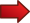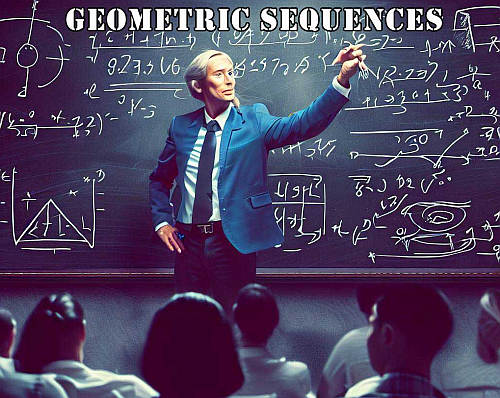# Geometric Sequences

A Geometric Sequence is a sequence of numbers that has the property that the ratio between two consecutive elements is constant, equal to a certain value $$r$$. This value is also known as the common ratio.

In a real life problem, you will be given an initial value $$a$$, and the constant ratio $$r$$ that is preserved between consecutive values in the sequence. Your task will be to compute the geometric sequence using this given information.

## How do I solve a geometric sequence?Assume that the first term is $$a$$. Then, the next term is $$a r$$, and the next is $$ar^2$$. And so on.

So, in other words, we start with the first term $$a$$, and the next term is always found by multiplying the previous term by $$r$$.So the first term is $$a_1 = a$$.The second term is $$a_2 = a r$$.The third term is $$a_3 = a r^2$$.

## Geometric sequence formula

By looking at the above example, what happens is that is initial value $$a$$ is multiplied by an extra $$r$$ at each step. Therefore, the general n th term is

$\large a_n = a r^{n-1}$

This means that after going forward $$n$$ steps, we get that the corresponding number in the sequence is $$a_n = a r^{n-1}$$. This is the formula for the geometric series pattern, and all you need is to plug in the values of $$a$$ and $$n$$ in the formula.

## So, how do you find the nth term in a geometric sequence?

Summarizing, in order to find the nth term in a geometric sequence, you need two pieces of information to define a geometric sequence: You need the initial term $$a$$, and the constant ratio $$r$$.

Then, the consecutive terms of the geometric sequence are obtained by multiplying the previous term by $$r$$. For example, 3, 6, 12, 24, ... is a geometric sequence since the initial value is $$a = 3$$ and then each subsequent value is obtained by multiplying the previous value by $$r = 2$$.

Also, for example, you can ask yourself what is the rule for 1 2 4 8 16, and whether it is geometric sequence. Well, we have that the initial value is $$a = 1$$, and each next value is obtained by multiplying the previous value by $$r = 2$$.### EXAMPLE 1: Example of a geometric sequence

Find the 6th term of a geometric sequence with initial term $$10$$, and $$r = 1/2$$.

So, how do you calculate a geometric sequence? Based on the information provided, we have enough information to define the geometric sequence. Indeed, we have the first term $$a = 10$$, and we have the constant ratio $$r = 1/2$$.

The general n th term is

$\large a_n = a r^{n-1}$

so then the 6 th term is

$\large \displaystyle a_{10} = a r^{6-1} = 10 \left(\frac{1}{2}\right)^5$ $\large = \frac{10}{32} = \frac{5}{16}$## Can the Common Ratio be Negative?

Yes, absolutely. The constant ratio $$r$$ can be negative. For example, we can have a geometric sequence with initial term $$a_1 = 1$$ and constant ratio $$r = -2$$. So then, the second term is $$a_2 = 1 \cdot (-2) = -2$$, $$a_3 = (-2) \cdot (-2) = 4$$, and so on.

So, it is the exactly the same rule: in order to the following term, we multiply the previous term by the constant ratio $$r$$, even if the constant ratio is negative.

### EXAMPLE 2

Find the 5th term of a geometric sequence with initial term $$3$$, and $$r = -2$$.

We have enough information to define the geometric sequence, because we have the first term $$a_1 = 3$$, and we have the constant ratio $$r = -2$$.

The general n th term (with negative constant ratio) is

$\large a_n = a r^{n-1} = 3 \cdot (-2)^{n-1}$

so then the 5 th term is

$\large \displaystyle a_{5} = a r^{5-1} = 3 \cdot (-2)^{5-1} = 3 \cdot (-2)^4 = 3 \cdot 16 = 48$

You can use our geometric sequence formula calculator to double check what you have found above, which is a explicit formula calculator.

### EXAMPLE 3

Consider the sequence 1, 1/2, 1/4, 1/16, ... Is this sequence geometric?

In order for a given sequence to be geometric, the terms need to have a common ratio. In this case, dividing the second term by the first term we get $$(1/2)/1 = 1/2$$.

Then, if we divide the third by the second term: $$(1/4)/(1/2) = 1/2$$. So far so good.

Now, if we divide the fourth by the third term: $$(1/16)/(1/4) = 1/4$$. It fails. It is not a geometric series, because it does not have a common ratio (the ratio is 1/2 for the first two terms, but then it is 1/4, so it is not constant).

Hence, the sequence is NOT a geometric sequence.

## More About the Geometric Sequences

The punchline you need to keep in mind. What is the formula of geometric sequence? Simple

$\large a_n = a r^{n-1}$

where $$a$$ is the initial term and $$r$$ is the constant ratio (or common ratio, as it is also called).

There are a couple of calculators that you may want to use that are related to the concept of geometric sequence, or geometric progression, as it is also called.

• First you can check our infinite geometric series sum calculator , which sums infinite terms of a geometric sequence. This sum will be well defined (converge) if the constant ratio is such that $$|r| < 1$$.

• Also, you will want to use our geometric sequence sum calculator , which computes the sum of terms in a geometric sequence, UP to a certain finite value. This sum is well defined without conditions on the constant ratio $$r$$, provided that we add up to a finite term of the sequence.

### Can a geometric sequence have a common ratio of 1?

Absolutely. The general term for a geometric sequence with a common ratio of 1 is

$\large a_n = a r^{n-1}= a \cdot 1^{n-1} = a$

So, a sequence with common ratio of 1 is a rather boring geometric sequence, with all the terms equal to the first term.